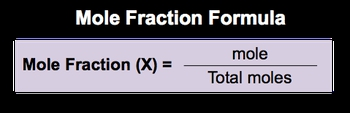## General Chemistry

Learn the toughest concepts covered in Chemistry with step-by-step video tutorials and practice problems by world-class tutors

7. Gases

# Mole Fraction -

Mole Fraction (X) represents the mole component by total moles.

Calculating Mole Fraction
1
concept

## Mole Fraction36s
Play a video:
Now, the concept of the mole fraction, which is represented by the variable X, represents the mold component divided by your total moles. Now, more component itself represents the element or compound. Who's more fraction You must determine taking this information. We now have our mole fraction formula. So mole fraction again, which is X equals your mole component. So what, they're asking to find the mole fraction off divided by your total moles. Now that we know the formula for mole fraction, continue on to the next video and let's take a look at an example question.2
example

## Mole Fraction Example 13m
Play a video: